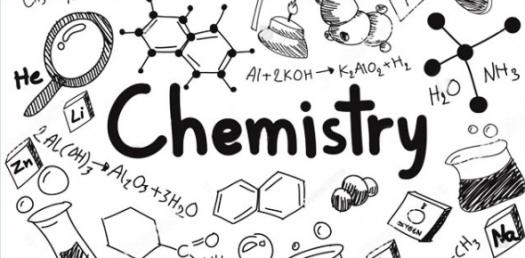# Grade 10 Questions On Atomic Structure

15 Questions | Attempts: 2295
ShareSettingsGrade 10 questions on atomic structure.

• 1.
What is the atomic number for hydrogen?
• A.

1

• B.

2

• C.

3

• D.

4

• E.

5

• 2.
What is the chemical symbol for calcium?
• A.

Cl

• B.

C

• C.

Ca

• D.

Cu

• E.

Cr

• 3.
How many neutrons are in nickel?
• A.

3

• B.

8

• C.

31

• D.

28

• E.

24

• 4.
How many protons are in nickel?
• A.

3

• B.

8

• C.

31

• D.

28

• E.

24

• 5.
What is the atomic mass of phosphorus?
• A.

15

• B.

31

• C.

2

• D.

18

• E.

16

• 6.
Which element has a mass of 65?
• 7.
What type of element is Potassium?
• A.

Metal

• B.

Non-metal

• C.

Metalloid

• D.

Noble Gas

• E.

Halogen

• 8.
The Lewis Dot diagram for Carbon would have how many dots (valence electrons)?
• A.

2

• B.

3

• C.

4

• D.

5

• E.

6

• 9.
What is Californium's atomic number?
• A.

96

• B.

97

• C.

98

• D.

99

• E.

100

• 10.
What is Californium's atomic mass?
• A.

247

• B.

247

• C.

251

• D.

252

• E.

257

• 11.
How many protons does Californium have?
• A.

96

• B.

97

• C.

99

• D.

98

• E.

100

• 12.
How many electrons does Californium have?
• A.

96

• B.

92

• C.

97

• D.

98

• E.

99

• 13.
How many netrons does Californium have?
• A.

110

• B.

153

• C.

98

• D.

251

• E.

1

• 14.
How many neutrons does Be have?
• A.

4

• B.

5

• C.

6

• D.

7

• E.

9

• 15.
What is the formula for calculating the number of neutrons in an element?
• A.

Atomic mass - atomic number

• B.

Atomic number - atomic mass

• C.

Atomic weight - atomic mass

• D.

Atomic mass - atomic weight

• E.

# of protons - # of electrons

## Related TopicsBack to top
×

Wait!
Here's an interesting quiz for you.